Question

# In java, write a program that gets 10 integer numbers from the user using user input,...

In java, write a program that gets 10 integer numbers from the user using user input, and then calculates and display the sum of the numbers that have been read.

Program Requirements:

Write the program in three versions with three loops. Put all three loops in the main method of your source code.

1. version1:  use a while loop.
2. version2:  use a do-while loop.
3. version 3:  use a for loop.

For each version, use a loop to input 10 int numbers from the user and calculate the sum.  Then display the sum on the console window.

CODE :

VERSION 1: Using For loop

import java.util.Scanner;

public class Main
{
public static void main(String[] args) {
Scanner sc = new Scanner(System.in);

//sum to store total sum of 10 integers
int sum=0,num;

System.out.println("Enter 10 integers : ");

//For loop
for(int i=0;i<10;i++){
//input of num
num = sc.nextInt();

sum = sum + num;

}

//printing sum
System.out.println("Sum = "+sum);
}
}
VERSION 1: Using While loop

import java.util.Scanner;

public class Main
{
public static void main(String[] args) {
Scanner sc = new Scanner(System.in);

//sum to store total sum of 10 integers
int sum=0,num;

System.out.println("Enter 10 integers : ");

//to count the number of integers input
int i=0;

//while loop
while(i<10){
//input of num
num = sc.nextInt();

sum = sum + num;

i++;
}

//printing sum
System.out.println("Sum = "+sum);
}
}
VERSION 1: Using Do While loop

import java.util.Scanner;

public class Main
{
public static void main(String[] args) {
Scanner sc = new Scanner(System.in);

//sum to store total sum of 10 integers
int sum=0,num;

System.out.println("Enter 10 integers : ");

//to count the number of integers input
int i=0;

//Do while loop
do{
//input of num
num = sc.nextInt();

sum = sum + num;

i++;

}while(i<10);

//printing sum
System.out.println("Sum = "+sum);
}
}

OUTPUT: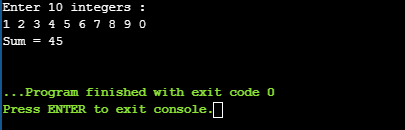#### Earn Coins

Coins can be redeemed for fabulous gifts.

Similar Homework Help Questions
• ### Using java Sample Outputs Write a program which asks the user for an integer and then...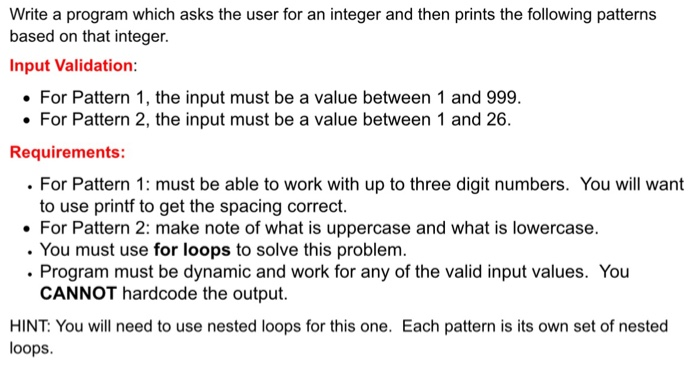Using java Sample Outputs Write a program which asks the user for an integer and then prints the following patterns based on that integer. Input Validation For Pattern 1, the input must be a value between 1 and 999 For Pattern 2, the input must be a value between 1 and 26. Requirements: For Pattern 1: must be able to work with up to three digit numbers. You will want to use printf to get the spacing correct. For Pattern...

• ### In this exercise, write a complete Java program that reads integer numbers from the user until...

In this exercise, write a complete Java program that reads integer numbers from the user until a negative value is entered. It should then output the average of the numbers, not including the negative number. If no non-negative values are entered, the program should issue an error message. You are required to use a do-while loop to solve this problem. Solutions that do not use a do-while loop will be given a zero. Make sure to add appropriate comments to...

• ### Write a program that receives a positive integer n from the user and then calculates the...

Write a program that receives a positive integer n from the user and then calculates the sum below by using a loop. Your program needs to ask the user to re-enter a different number if the user enters a non-positive one. Display the result. 1 SUM 1 2 3 ... n x x n = = = + + + +

• ### 21 Write a program that asks the user to input the length and breadth of a...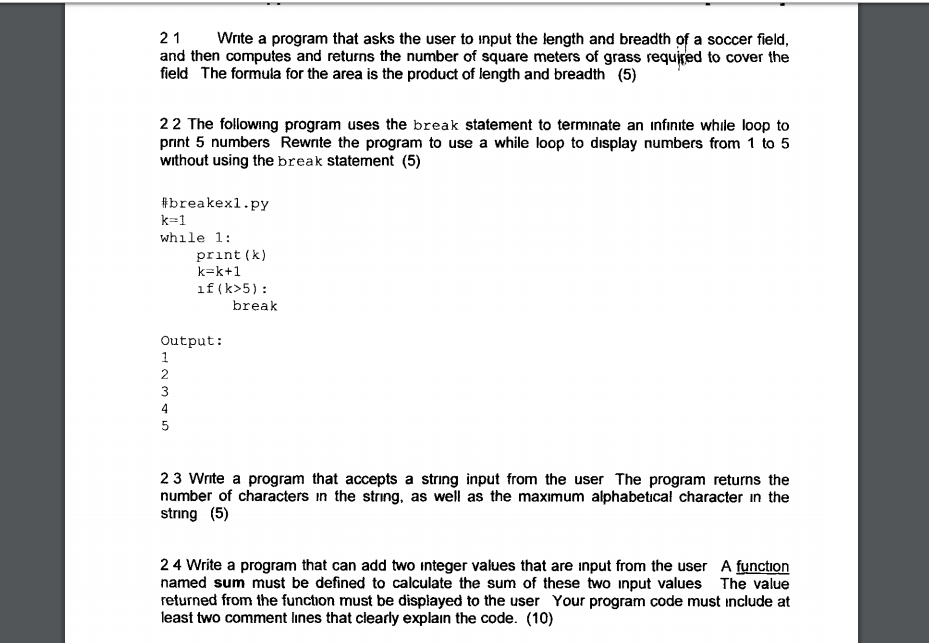21 Write a program that asks the user to input the length and breadth of a soccer field, and then computes and returns the number of square meters of grass required to cover the field The formula for the area is the product of length and breadth (5) 22 The following program uses the break statement to terminate an infinite while loop to print 5 numbers Rewrite the program to use a while loop to display numbers from 1 to...

• ### Using C++ Reference Parameters Assignment Write a program that asks the user for 3 numbers, sends...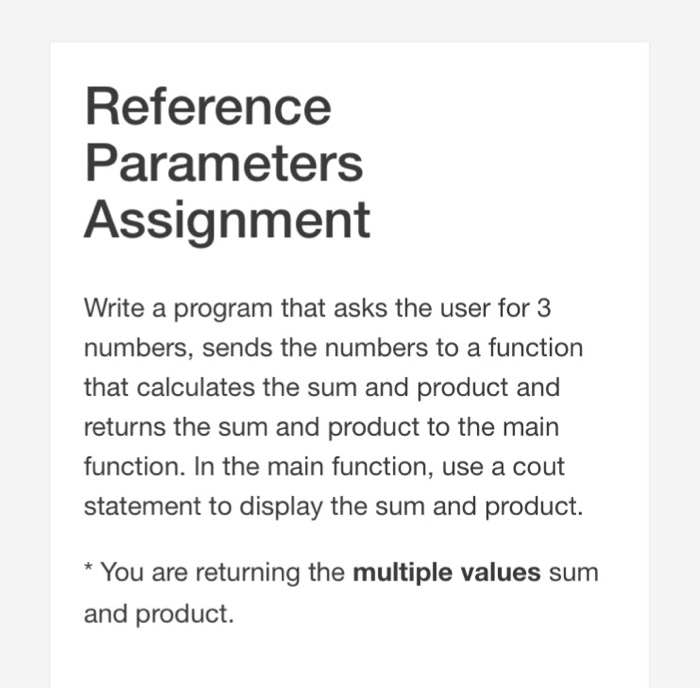Using C++ Reference Parameters Assignment Write a program that asks the user for 3 numbers, sends the numbers to a function that calculates the sum and product and returns the sum and product to the main function. In the main function, use a cout statement to display the sum and product. * You are returning the multiple values sum and product.

• ### Lab 5-2 Nested Loops 2. Summation Of Numbers (using Nested While Loops) Part A: The program...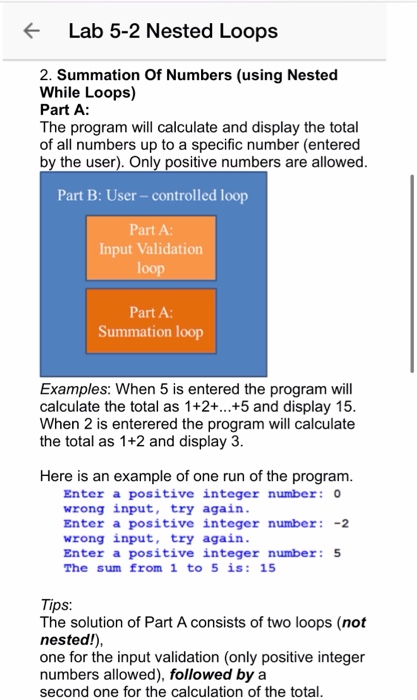Lab 5-2 Nested Loops 2. Summation Of Numbers (using Nested While Loops) Part A: The program will calculate and display the total of all numbers up to a specific number (entered by the user). Only positive numbers are allowed. Part B: User-controlled loop Part A Input Validation loop Part A: Summation loop Examples: When 5 is entered the program will calculate the total as 1+2+...+5 and display 15. When 2 is enterered the program will calculate the total as 1+2...

• ### Task 1 : Write a Java program that prompts for and reads 10 integers from the...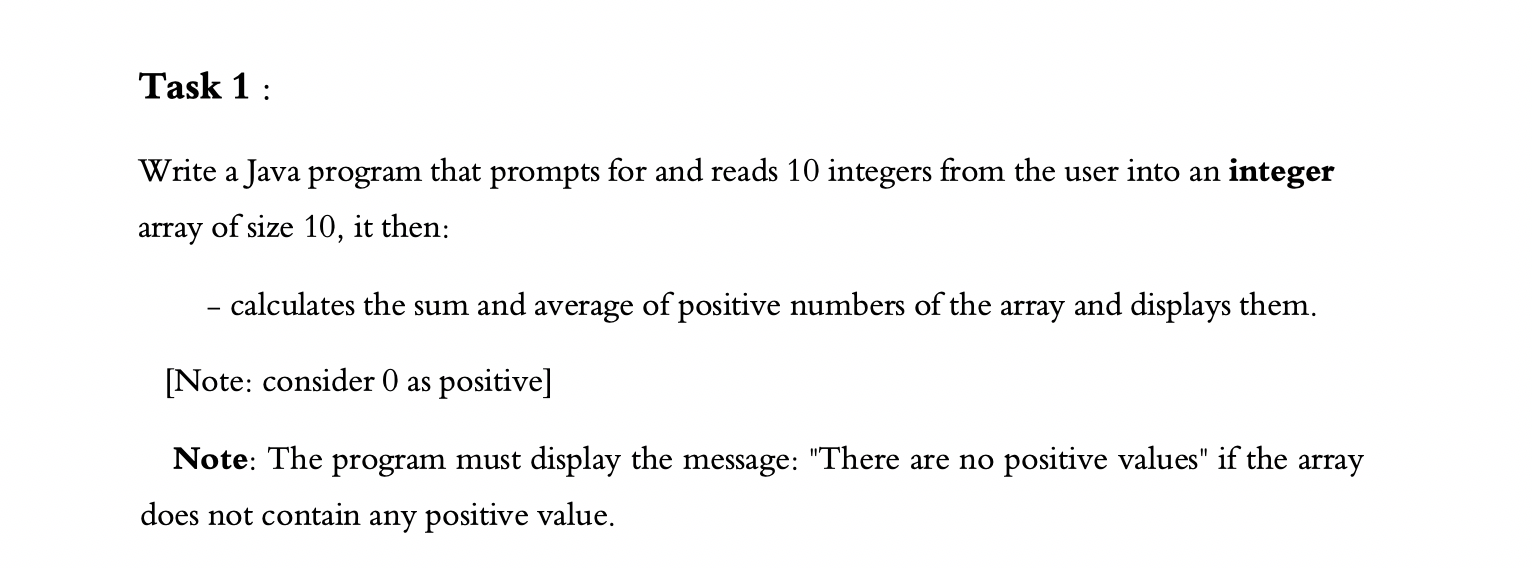Task 1 : Write a Java program that prompts for and reads 10 integers from the user into an integer array of size 10, it then: - calculates the sum and average of positive numbers of the array and displays them. [Note: consider 0 as positive] Note: The program must display the message: "There are no positive values" if the array does not contain any positive value.

• ### Please write a java program that takes in user input of various numbers as a type...

Please write a java program that takes in user input of various numbers as a type string, prints the sum of all numbers entered, and prints the smallest and largest numbers. Arrays are optional as they have not been covered by my professor yet. Thank you

• ### java programe Write a program that prompts the user to enter in an integer number representing...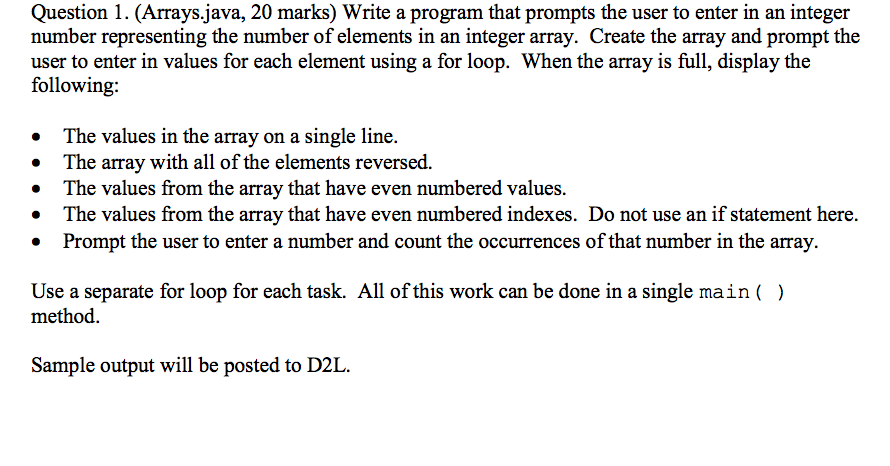java programe Write a program that prompts the user to enter in an integer number representing the number of elements in an integer array. Create the array and prompt the user to enter in values for each element using a for loop. When the array is full, display the following: The values in the array on a single line. The array with all of the elements reversed. The values from the array that have even numbered values. The values from...

• ### Write a program that calculates the average of a stream of non-negative numbers. The user can...

Write a program that calculates the average of a stream of non-negative numbers. The user can enter as many non-negative numbers as they want, and they will indicate that they are finished by entering a negative number. For this program, zero counts as a number that goes into the average. Of course, the negative number should not be part of the average (and, for this program, the average of 0 numbers is 0). You must use a method to read...